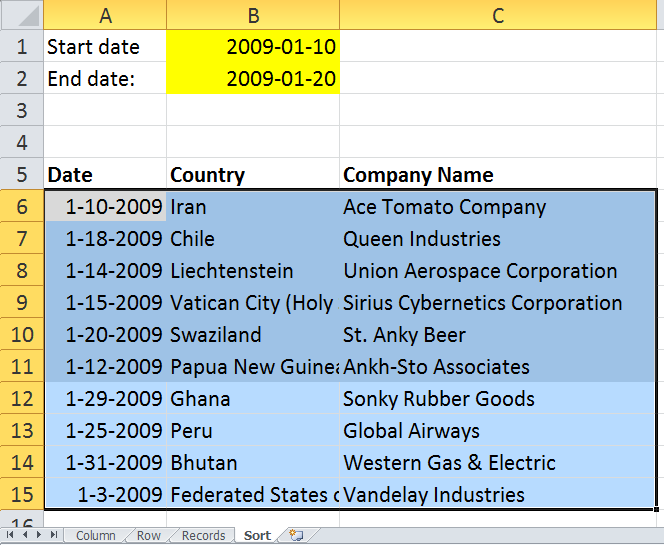Author: Oscar Cronquist Article last updated on November 17, 2018

Question: How do I highlight dates that meet criteria using conditional formatting?

Highlight values in a column

Highlight values in a row

Highlight records

Sort values

### Highlight values in a column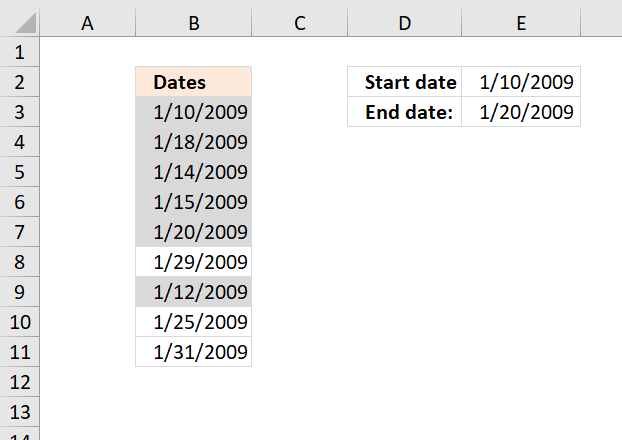### How to apply the conditional formatting formula

1. Select the range (B3:B11)
2. Click "Home" tab on the ribbon
3. Click "Conditional formatting"
4. Click "New rule..."
5. Click "Use a formula to determine which cells to format"
6. Click "Format values where this formula is true" window.
7. Type
=(B3<=\$E\$3)*(B3>=\$E\$2)
8. Click Format button
9. Click "Fill" tab
10. Select a color
11. Click OK!
12. Click OK!

### Explaining CF formula

B3<=\$E\$2

becomes

39823<=39833

and returns TRUE

#### Step 2 - Check if current cell date is larger than or equal to start date

B3>=\$E\$2

becomes

39823>=39823

and returns TRUE.

#### Step 3 - Multiply boolean values

Both boolean values must be TRUE in order to highlight the cell.

 Boolean Boolean Multiply Add FALSE FALSE 0  (zero) 0 (zero) FALSE TRUE 0  (zero) 1 TRUE TRUE 1 2

The parentheses make sure that the order of calculation is correct.

(B3<=\$E\$3)*(B3>=\$E\$2)

becomes

TRUE*TRUE

and returns 1 which is equivalent to TRUE. Cell B3 is highlighted.

### Highlight values in a row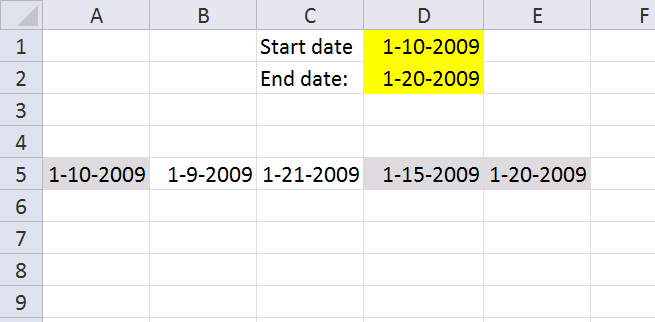Conditional formatting formula in cell range A5:E5:

=(A5<=\$D\$2)*(A5>=\$D\$1)

This formula is the same as the above (not the cell references though), the difference is that it is applied to a horizontal cell range.

### Highlight records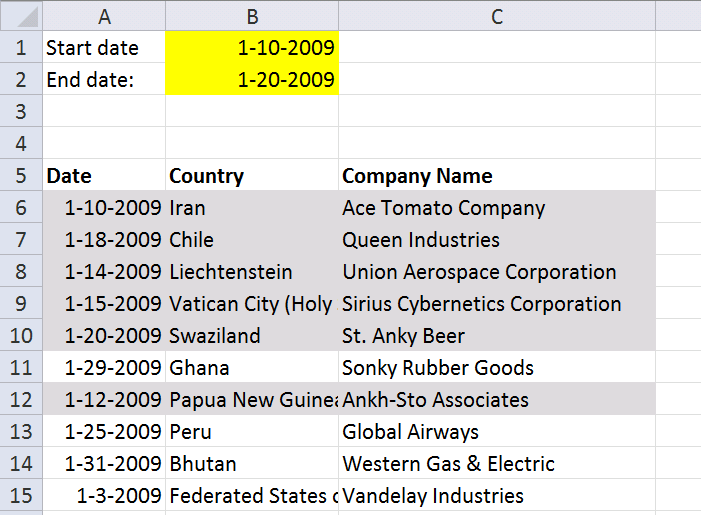Conditional formatting formula in cell range A6:C15:

=(\$A6<=\$B\$2)*(\$A6>=\$B\$1)

The cell references are "locked" to column A, that is why there is a dollar sign in front of \$A6. That makes the entire row highlighted if the date cell is in the date range.

### Sort values

You can quickly sort highlighted records to the top of the list.

1. Select cell range A6:C15
2. Right click on cell range
3. Click "sort"
4. Click "Put selected cell color on top"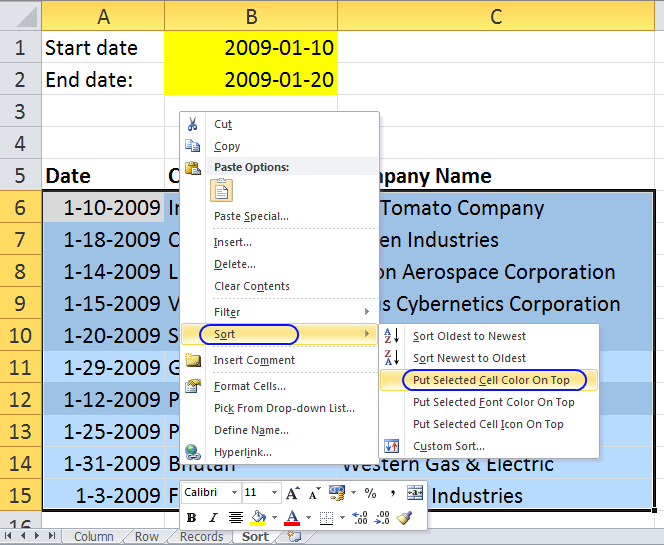All highlighted records are on top.